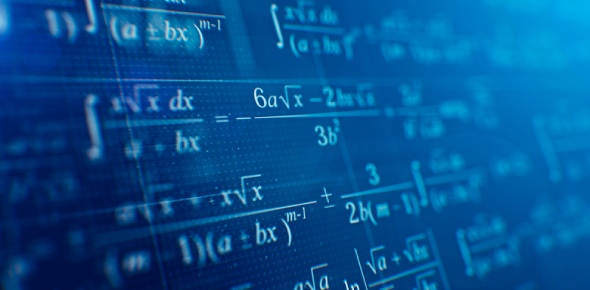# Quiz: Mathematics Ss1 First Term

20 Questions | Total Attempts: 2685SettingsThink you are a mathematics expert? Wanna prove your knowledge? This on mathematics quiz may be a challenge for you. This quiz wants you to know how to make a letter the subject of the formula of the relation, find the median, how to solve for x, and which set contains all the possible elements under consideration. This quiz is for individuals who wish to test themselves in the area of mathematics.

• 1.
Make w the subject of the formula of the relation (a + bc)/(wd + f)= g
• A.

(a + bc - fg)/dg

• B.

(a - bc + fg)/dg

• C.

(a + bc - f)/dg

• D.

(a + bc - dg)/fg

• 2.
The term that refers to the relationship between two or more quantities in which a change in one quantity result in a change in the other(s) is called:
• A.

Variation

• B.

Variance

• C.

Determinant

• D.

Algebraic fractions

• 3.
Find the median of 2, 1, 0, 3, 1, 1, 4, 0, 1 and 2.
• A.

0.0

• B.

0.5

• C.

1.0

• D.

1.5

• 4.
Solve for x, if 5x = 5√5.
• A.

31/2

• B.

11/2

• C.

3/4

• D.

1/4

• 5.
The mean of numbers 4, 6, 4, 7, (x + 1), 8 and 2 is 5 find the median of the numbers:
• A.

4

• B.

4.5

• C.

5

• D.

Option 4

• 6.
Simplify 8n × 22n ÷ 43n
• A.

2(-n)

• B.

2(1-n)

• C.

2n

• D.

2(n+1)

• 7.
Given that 2x= 0.125, find the value of x.
• A.

0

• B.

-1

• C.

-2

• D.

-3

• 8.
Solve for x: x2 + 2x + 1 = 25
• A.

-6,-4

• B.

6,-4

• C.

6,4

• D.

-6,4

• 9.
Convert 8910 to a number in base two.
• A.

1101001

• B.

1011001

• C.

1001101

• D.

101101

• 10.
Solve the simultaneous equation y =3x, 4y – 5x = 14
• A.

-2, -6

• B.

2,6

• C.

2,-6

• D.

-1,-3

• 11.
Which of the following is equal to 72/125:
• A.

(23 × 32)/53

• B.

(24 x 33)/53

• C.

(24 × 3)/53

• D.

(24 × 32)/53

• 12.
If R = {2, 4, 6, 7} and S = {1, 2, 4, 8}, then R∪S equals?
• A.

{2, 4}

• B.

{1, 2, 4, 7, 8}

• C.

{2, 6, 7}

• D.

{1, 2, 4, 6, 7, 8}

• 13.
Simplify 15x2y3z ÷ 3x2yz-2
• A.

5xy2z

• B.

5x2yz

• C.

5x2z

• D.

5y2z3

• 14.
If U = (all letters of alphabet), A ={f, a, k, e} (b.) {s, p, e, a, k} the A ∩ B = ?
• A.

{a,e,k}

• B.

{s,e,k}

• C.

{f,e, k}

• D.

{p,e,k}

• 15.
The set which contains all possible elements under consideration is ………
• A.

Super set

• B.

Null set

• C.

Universal set

• D.

Finite set

• 16.
The relationship consisting of two or more parts added together is called partial variation.
• A.

True

• B.

False

• 17.
The set of prime numbers is …
• A.

1,3,5

• B.

2,3,5

• C.

2,4,6

• D.

3, 9,15

• 18.
Correct 0.04945 to two significant figures:
• A.

0.040

• B.

0.049

• C.

0.0495

• D.

0.050

• 19.
Express 0.00562 in standard form:
• A.

0.562 x 10-3

• B.

5.62 x 10-2

• C.

5.62 x10-3

• D.

5.62 x 103

• 20.
Change 32five to a given number in base three (A) 221three      (B) 122three         (C) 121three           (D) 101three
• A.

Option 1

• B.

Option 2

• C.

Option 3

• D.

Option 4

Related TopicsBack to top